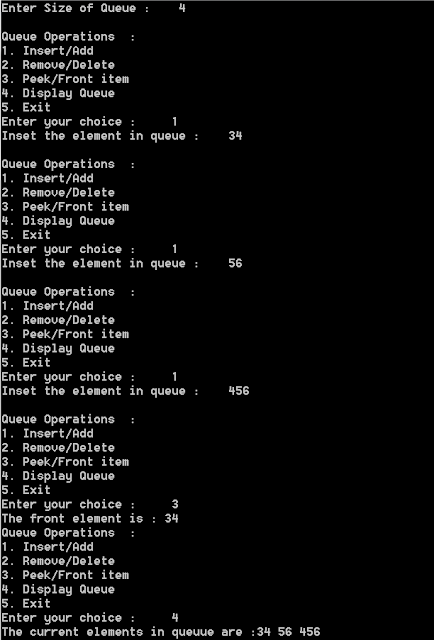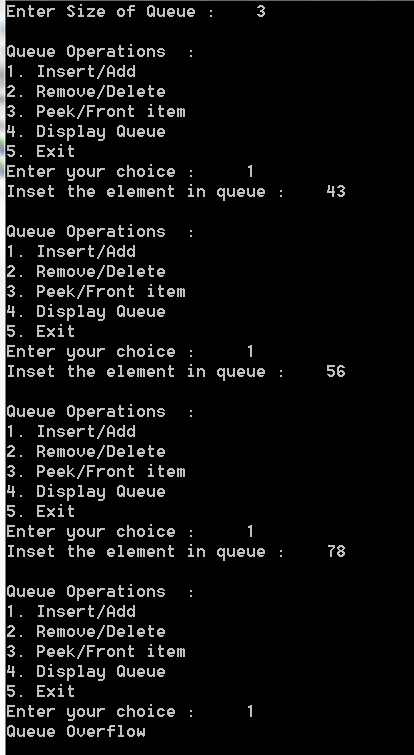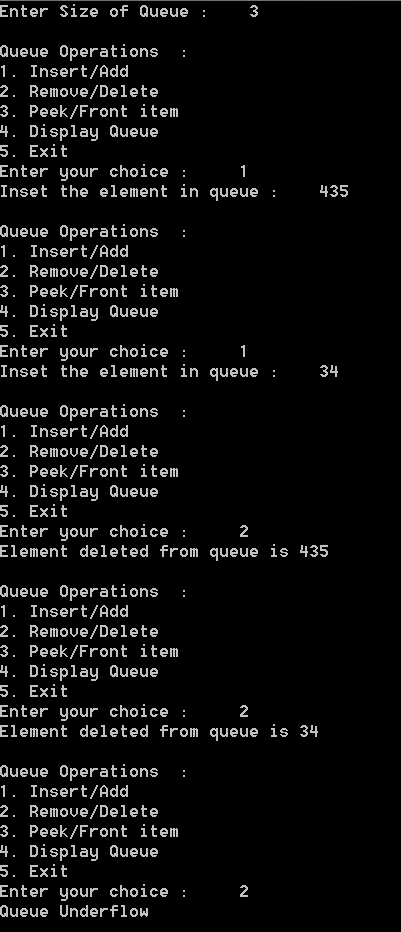## Tuesday, 16 February 2016

### Data Structures - Cpp Program to implement queue operations using an array

Queue is a data structure  , similar to Stack , but here in queue we have openings at both the ends , one for insertion and other for deletion.

 Some of the common operations of queue are : Adding an item to queue(enqueue) Removing an item from a queue(dequeue) Overflow Condition(Queue full) Underflow Condition(Queue empty) Getting the front element(peek)

Overflow situation occur when the queue is full and then we are trying to  insert/add another item to the queue .

Underflow situation occur when the queue is empty and the we are trying to remove/delete an item from the queue.

The principle of  queue is First In First Out (FIFO).

Download code

## PROGRAM :

```#include <iostream>

using namespace std;

int main()
{
int rear = -1,front = -1,item,MAX ,choice ,i;
cout << "Enter Size of Queue : \t ";
cin >> MAX;
int QueueArray[MAX];
while (1)
{
cout << "\nQueue Operations  :";
cout << "\n1. Insert/Add";
cout << "\n2. Remove/Delete";
cout << "\n3. Peek/Front item";
cout << "\n4. Display Queue";
cout << "\n5. Exit";
cout << "\nEnter your choice :\t";
cin >> choice;
switch (choice)
{
case 1:
if (rear == MAX - 1)
{
cout << "Queue Overflow \n";
}
else
{
if (front == -1)
{
front = 0;
}
cout << "Inset the element in queue : \t";
cin >> item;
rear = rear + 1;
QueueArray[rear] = item;
}

break;
case 2:

if (front == -1 || front > rear)
{
cout << "Queue Underflow \n";
break;
}
else
{
cout << "Element deleted from queue is " <<  QueueArray[front] << "\n" ;
front = front + 1;
}
break;
case 3:
if (front == -1 || front > rear)
{
cout << "Queue Underflow \n";
break;
}
else
{
cout << "The front element is :" << QueueArray[front] << "\t" ;
}
break;
case 4:
if (front == -1 || front > rear)
{
cout << "Queue Underflow \n";
break;
}
else
{
cout << "The current elements in queue are :";
for (i = front; i <= rear; i++)
{
cout << QueueArray[i];
}
}
break;
case 5:
return 0;
break;
default:
cout << "Wrong Entry \n ";
break;
}

}
return 0;
}
```
Download code

## OUTPUT :Cpp - Queue OperationsCpp- Queue OverflowCpp - Queue Underflow
Download code